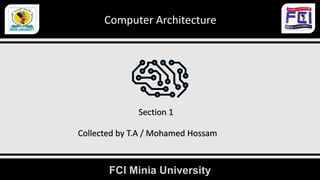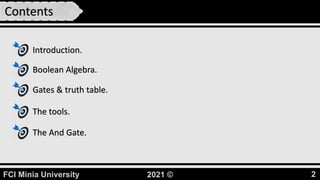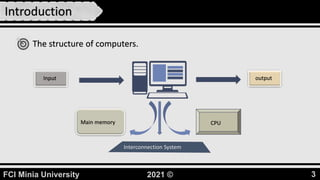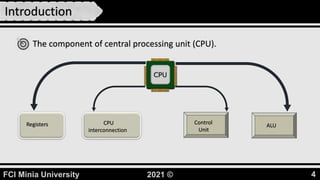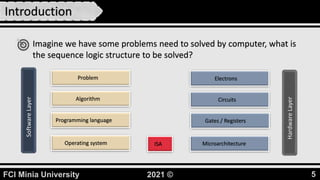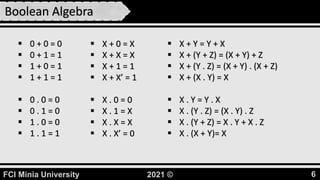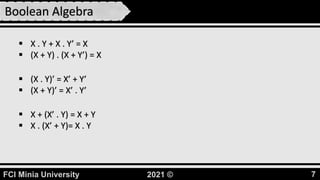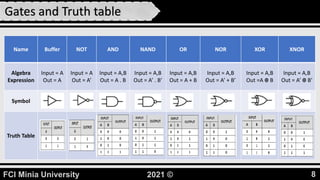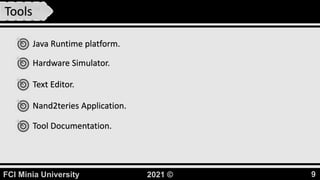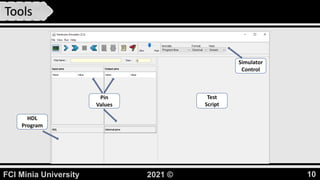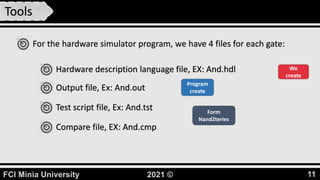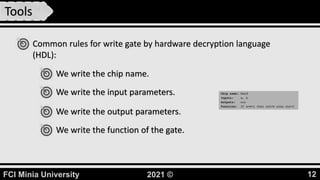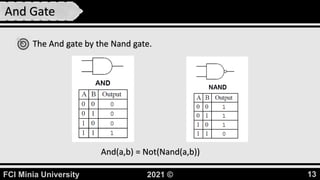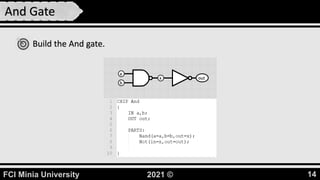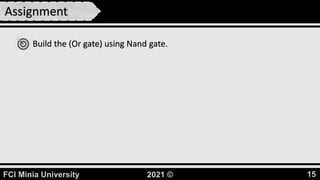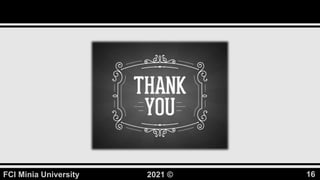1 of 16

### HDL.pptx

1. FCI Minia University Computer Architecture Section 1 Collected by T.A / Mohamed Hossam
2. FCI Minia University 2021 © 2 Contents Introduction. Boolean Algebra. Gates & truth table. The tools. The And Gate.
3. FCI Minia University 2021 © 3 Introduction The structure of computers. Input output CPU Main memory Interconnection System
4. FCI Minia University 2021 © 4 Introduction The component of central processing unit (CPU). Registers CPU interconnection Control Unit ALU
5. FCI Minia University 2021 © 5 Introduction Imagine we have some problems need to solved by computer, what is the sequence logic structure to be solved? Software Layer Problem Algorithm Programming language Operating system Hardware Layer Microarchitecture Gates / Registers Circuits Electrons ISA
6. FCI Minia University 2021 © 6 Boolean Algebra  0 + 0 = 0  0 + 1 = 1  1 + 0 = 1  1 + 1 = 1  0 . 0 = 0  0 . 1 = 0  1 . 0 = 0  1 . 1 = 1  X + 0 = X  X + X = X  X + 1 = 1  X + X’ = 1  X . 0 = 0  X . 1 = X  X . X = X  X . X’ = 0  X + Y = Y + X  X + (Y + Z) = (X + Y) + Z  X + (Y . Z) = (X + Y) . (X + Z)  X + (X . Y) = X  X . Y = Y . X  X . (Y . Z) = (X . Y) . Z  X . (Y + Z) = X . Y + X . Z  X . (X + Y)= X
7. FCI Minia University 2021 © 7 Boolean Algebra  X . Y + X . Y’ = X  (X + Y) . (X + Y’) = X  X + (X’ . Y) = X + Y  X . (X’ + Y)= X . Y  (X . Y)’ = X’ + Y’  (X + Y)’ = X’ . Y’
8. FCI Minia University 2021 © 8 Gates and Truth table Name Buffer NOT AND NAND OR NOR XOR XNOR Algebra Expression Input = A Out = A Input = A Out = A’ Input = A,B Out = A . B Input = A,B Out = A’ . B’ Input = A,B Out = A + B Input = A,B Out = A’ + B’ Input = A,B Out =A Ꚛ B Input = A,B Out = A’ Ꚛ B’ Symbol Truth Table
9. FCI Minia University 2021 © 9 Tools Java Runtime platform. Hardware Simulator. Text Editor. Nand2teries Application. Tool Documentation.
10. FCI Minia University 2021 © 10 Tools Pin Values HDL Program Test Script Simulator Control
11. FCI Minia University 2021 © 11 Tools For the hardware simulator program, we have 4 files for each gate: Hardware description language file, EX: And.hdl Test script file, Ex: And.tst Output file, Ex: And.out We create Program create Compare file, EX: And.cmp Form Nand2teries
12. FCI Minia University 2021 © 12 Tools Common rules for write gate by hardware decryption language (HDL): We write the chip name. We write the input parameters. We write the output parameters. We write the function of the gate.
13. FCI Minia University 2021 © 13 And Gate The And gate by the Nand gate. And(a,b) = Not(Nand(a,b))
14. FCI Minia University 2021 © 14 And Gate Build the And gate. a b x out
15. FCI Minia University 2021 © 15 Assignment Build the (Or gate) using Nand gate.
16. FCI Minia University 2021 © 16

### Editor's Notes

1. Arithmetic and Login Unit (ALU).
2. Instruction Set Architecture (ISA): - is interface layer to communicate between the software and hardware layer.
3. Prove the (X . (X + Y)= X) or (X + (X . Y) = X).
4. Nand2teries Application have all gates built in functions and compare file to test the results.
5. Hardware Description Language (HDL).
6. We build all gates by the built in gates (Nand).